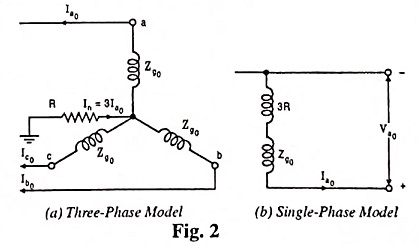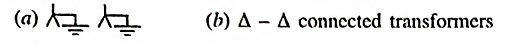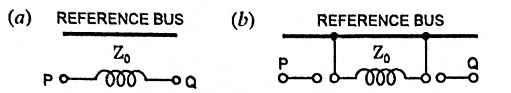## Symmetrical Components Interview Questions and Answers:

1. What do you mean by Fortescue theorem.

Ans. According to Fortescue theorem, any unbalanced three-phase system of currents, voltages or other sinusoidal quantities can be resolved into three balanced systems of phasors which are called symmetrical components of the original unbalanced system. Such three balanced systems constitute three sequence networks which are solved separately on a single phase basis.

2. What are symmetrical components ?

Ans. According to the theory of symmetrical components, any unbalanced system of phasors (voltages, currents or impedances etc.) can be resolved into balanced systems of phasors equal in number to the number of phases. These balanced systems of phasors are called the symmetrical components of the original unbalanced system.

3. What are the uses of symmetrical components in power systems ?

Ans. Symmetrical component method provides a useful analytical tool for the protection engineer and is useful in system fault calculation and system stability problems etc.

4. Differentiate between the positive sequence system and zero sequence system.

Ans. The positive sequence system is a set of three symmetrical voltages (i.e., all numerically equal and all displaced from each other by 120°) having the same phase sequence as the original set of three unbalanced voltages i.e., A-B-C whereas the zero sequence system is a set of three voltages, all equal in magnitude and in phase with each other.

5. Define negative sequence impedance.

Ans. The impedance of the network offered to the flow of negative sequence currents is called the negative sequence impedance of the network.

6. What are sequence networks ?

Ans. The single phase equivalent circuit composed of impedances to current of any one sequence only is called the sequence network for that particular sequence. Hence corresponding to positive, negative and zero sequence currents we have positive, negative and zero sequence networks.

7. Draw the zero sequence network for a star-connected generator earthed through a resistance R.

Ans.8. The neutral grounding impedance Zn appears as 3 Zn in the zero sequence equivalent circuit why ?

Ans. As the current flowing in the neutral grounding impedance Zn is the sum of the zero sequence currents in all the three phases, hence voltage drop caused by it will be 3Ia0 Zn. That is why neutral grounding impedance Zn appears as 3 Zn in the zero sequence equivalent circuit.

9. Draw the zero sequence networks forAns.10. Draw zero sequence impedance networks for the below three-phase transformer connections.Ans. Zero sequence equivalent circuits are given below :

Scroll to Top